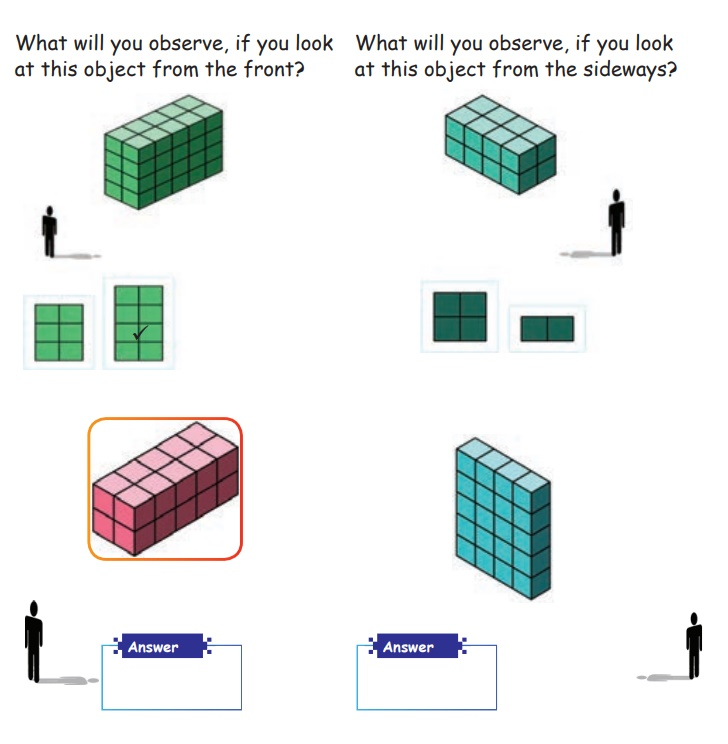Home | | Maths 5th Std | Draw 3-D shapes from 2-D Shapes

# Draw 3-D shapes from 2-D Shapes

Properties/characteristics and Examples of Cone, Sphere, Cylinder, Cuboid, Cube.

Draw 3-D shapes from 2-D Shapes

Cube

Properties/characteristics:

* It is a 3-D shape

* It has six faces

* All sides are equal.

* It has 8 vertices and 12 edges.Examples:Cuboid

Properties/Characteristics

* It is a 3-D shape

* It has six faces

* It‛s opposite sides are equal

* It has 8 vertices and 12 edges.

Examples:Cylinder

Properties/Characteristics

* It is a 3-D shape

* Two bases lie in upper and lower surfaces in a cylinder.

* Height is the distance between the two bases.

Examples:Sphere

Properties/Characteristics

* It is a 3-D shape

* It has one surface

* All points on the surface are at the same distance from the center

* It has no vertices and edges

Examples:Cone

Properties/Characteristics

* It is a 3-D Shape.

* Base of a cone is circular.

* The distance from the top of the cone to the center of the base is called as height.

* The distance from the apex to any point lying on the circumference of base is called as slant height.

* The height and slant height are not equal.

Examples:l − slant height

h − height

Activity

Write the 3-D shapes lying around usWhat will you observe, if you look at this object from the front?

What will you observe, if you look at this object from the sideways?

Tags : Geometry | Term 1 Chapter 1 | 5th Maths , 5th Maths : Term 1 Unit 1 : Geometry
Study Material, Lecturing Notes, Assignment, Reference, Wiki description explanation, brief detail
5th Maths : Term 1 Unit 1 : Geometry : Draw 3-D shapes from 2-D Shapes | Geometry | Term 1 Chapter 1 | 5th Maths